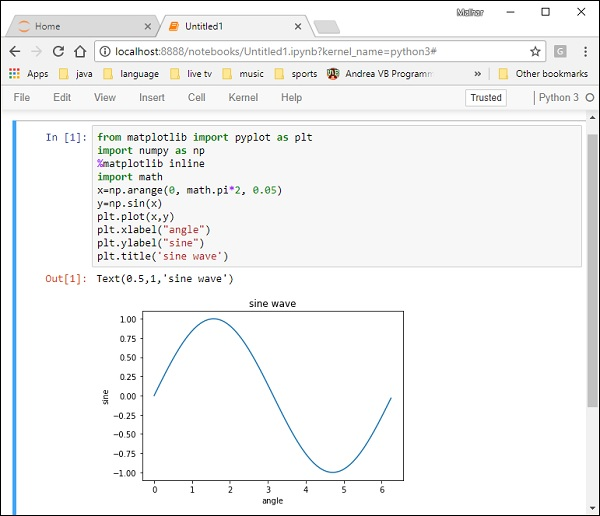# Matplotlib - Simple Plot

In this chapter, we will learn how to create a simple plot with Matplotlib.

We shall now display a simple line plot of angle in radians vs. its sine value in Matplotlib. To begin with, the Pyplot module from Matplotlib package is imported, with an alias plt as a matter of convention.

```import matplotlib.pyplot as plt
```

Next we need an array of numbers to plot. Various array functions are defined in the NumPy library which is imported with the np alias.

```import numpy as np
```

We now obtain the ndarray object of angles between 0 and 2π using the arange() function from the NumPy library.

```x = np.arange(0, math.pi*2, 0.05)
```

The ndarray object serves as values on x axis of the graph. The corresponding sine values of angles in x to be displayed on y axis are obtained by the following statement −

```y = np.sin(x)
```

The values from two arrays are plotted using the plot() function.

```plt.plot(x,y)
```

You can set the plot title, and labels for x and y axes.

```You can set the plot title, and labels for x and y axes.
plt.xlabel("angle")
plt.ylabel("sine")
plt.title('sine wave')
```

The Plot viewer window is invoked by the show() function −

```plt.show()
```

The complete program is as follows −

```from matplotlib import pyplot as plt
import numpy as np
import math #needed for definition of pi
x = np.arange(0, math.pi*2, 0.05)
y = np.sin(x)
plt.plot(x,y)
plt.xlabel("angle")
plt.ylabel("sine")
plt.title('sine wave')
plt.show()
```

When the above line of code is executed, the following graph is displayed −Now, use the Jupyter notebook with Matplotlib.

Launch the Jupyter notebook from Anaconda navigator or command line as described earlier. In the input cell, enter import statements for Pyplot and NumPy −

```from matplotlib import pyplot as plt
import numpy as np
```

To display plot outputs inside the notebook itself (and not in the separate viewer), enter the following magic statement −

```%matplotlib inline
```

Obtain x as the ndarray object containing angles in radians between 0 to 2π, and y as sine value of each angle −

```import math
x = np.arange(0, math.pi*2, 0.05)
y = np.sin(x)
```

Set labels for x and y axes as well as the plot title −

```plt.xlabel("angle")
plt.ylabel("sine")
plt.title('sine wave')
```

Finally execute the plot() function to generate the sine wave display in the notebook (no need to run the show() function) −

```plt.plot(x,y)
```

After the execution of the final line of code, the following output is displayed −